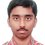# Number Theory problem

A four digit number has the following properties:

(i) it is a perfect square,

(ii) its first two digits are equal to each other,

(iii) its last two digits are equal to each other .

Find all such four-digit numbers.

Till now I have been able to find only one such number. I don't know if I am correct or not. Any idea ?Note by Nishant Sharma
6 years, 2 months ago

MarkdownAppears as
*italics* or _italics_ italics
**bold** or __bold__ bold
- bulleted- list
• bulleted
• list
1. numbered2. list
1. numbered
2. list
Note: you must add a full line of space before and after lists for them to show up correctly
paragraph 1paragraph 2

paragraph 1

paragraph 2

[example link](https://brilliant.org)example link
> This is a quote
This is a quote
    # I indented these lines
# 4 spaces, and now they show
# up as a code block.

print "hello world"
# I indented these lines
# 4 spaces, and now they show
# up as a code block.

print "hello world"
MathAppears as
Remember to wrap math in $ ... $ or $ ... $ to ensure proper formatting.
2 \times 3 $2 \times 3$
2^{34} $2^{34}$
a_{i-1} $a_{i-1}$
\frac{2}{3} $\frac{2}{3}$
\sqrt{2} $\sqrt{2}$
\sum_{i=1}^3 $\sum_{i=1}^3$
\sin \theta $\sin \theta$
\boxed{123} $\boxed{123}$

## Comments

Sort by:

Top Newest

Let the first two digits be $a$, and the last two digits be $b$. Then the number is $N = 1000a + 100a + 10b + b = 1100a + 11b= 11(100a+b)$. Note that $11$ divides $N$, and $11$ being a prime, for $N$ to be a perfect square $11$ must also divide $\frac{N}{11}$, i.e. $11$ must divide $100a + b$. But note that $100a + b= 99a + a+b = 9*11a + a+b$. Hence for $11$ to divide $100a + b$, $11$ must divide $a+b$, i.e. $a+b$ must be a multiple of $11$. Note that $1 \leq a \leq 9$ , $0 \leq b \leq 9$. Thus $1 \leq a+b \leq 18$. The only multiple of $11$ between $1$ and $18$ is $11$ itself. Thus $a+b= 11$. Now note that the quadratic residues modulo $10$ are $0$, $1$, $4$, $5$, $6$, and $9$. Thus the only possible values of $b$ are$4$, $5$, $6$, and $9$ (note that $b$ can't be $0$ or $1$ since that would imply $a= 11$ or $a= 10$ respectively ) .

For $b= 4$, we have $a= 7$, and the number is $7744 = 88^2$ (a perfect square). For $b= 5$ we have $a= 6$ and the number is $6655$ (not a perfect square). For $b= 6$ we have $a= 5$ and the number is $5566$ (not a perfect square). For $b= 7$ we have $a= 4$ and the number is $4477$ (not a perfect square).

Hence there is only one number that satisfies the given conditions and it is $7744$.

- 6 years, 2 months ago

Log in to reply

This is good, though there isn't a need to appeal to quadratic residues (for those who don't know it).

Once you know that the cases are $a+b = 11$, there are 9 values to check for squares, and this can be done easily.

Alternatively if you are too lazy to find square roots, note that $\overline{aabb} = 121 \times (9a + 1 )$ (using that $a+b = 11$ ), so $9a + 1$ must be a perfect square. Checking $a = 1$ to 9, gives $a = 7$ as the only square.

Staff - 6 years, 2 months ago

Log in to reply

I could understand everything except the "quadratic residues modulo 10" thing. Please explain what does that mean ?

- 6 years, 2 months ago

Log in to reply

It means the last digit of a perfect square number can only be 0,1,4,5,6,9. You can prove it yourself, it is quite easy. Best wishes :)

- 6 years, 2 months ago

Log in to reply

Oh I see.

- 6 years, 2 months ago

Log in to reply

An integer $q$ is called a quadratic residue mod $n$ if and only if there exists an integer $x$ such that $x^2 ≡ q (mod n)$. To put it other way round, if a sequence of integers $r_i$ is defined such that $r_i$ is the remainder when $i^2$ is divided by $n$, then the quadratic residues mod $n$ are the distinct elements of the set $[ r_1, r_2, ..., r_{n-1} ]$.

- 6 years, 2 months ago

Log in to reply

Isn't this an RMO problem?

- 6 years, 2 months ago

Log in to reply

Yep, RMO 1991 or 1992 maybe.

- 5 years, 11 months ago

Log in to reply

×

Problem Loading...

Note Loading...

Set Loading...